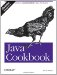# Recipe 5.21 Program: Number Palindromes

My wife, Betty, recently reminded me of a theorem that I must have studied in high school but whose name I have long since forgotten: that any positive integer number can be used to generate a palindrome by adding to it the number comprised of its digits in reverse order. Palindromes are sequences that read the same in either direction, such as the name "Anna" or the phrase "Madam, I'm Adam" (ignoring spaces and punctuation). We normally think of palindromes as composed of text, but the concept can be applied to numbers: 13531 is a palindrome. Start with the number 72, for example, and add to it the number 27. The results of this addition is 99, which is a (short) palindrome. Starting with 142, add 241, and you get 383. Some numbers take more than one try to generate a palindrome. 1951 + 1591 yields 3542, which is not palindromic. The second round, however, 3542 + 2453, yields 5995, which is. The number 17,892, which my son Benjamin picked out of the air, requires 12 rounds to generate a palindrome, but it does terminate:

`C:\javasrc\numbers>java  Palindrome 72 142 1951 17892 Trying 72 72->99 Trying 142 142->383 Trying 1951 Trying 3542 1951->5995 Trying 17892 Trying 47763 Trying 84537 Trying 158085 Trying 738936 Trying 1378773 Trying 5157504 Trying 9215019 Trying 18320148 Trying 102422529 Trying 1027646730 Trying 1404113931 17892->2797227972 C:\javasrc\numbers>`

If this sounds to you like a natural candidate for recursion, you are correct. Recursion involves dividing a problem into simple and identical steps, which can be implemented by a function that calls itself and provides a way of termination. Our basic approach, as shown in method findPalindrome, is:

`long findPalindrome(long num) {      if (isPalindrome(num))         return num;      return findPalindrome(num + reverseNumber(num)); }`

That is, if the starting number is already a palindromic number, return it; otherwise, add it to its reverse, and try again. The version of the code shown here handles simple cases directly (single digits are always palindromic, for example). We won't think about negative numbers, as these have a character at the front that loses its meaning if placed at the end, and hence are not strictly palindromic. Further, palindromic forms of certain numbers are too long to fit in Java's 64-bit long integer. These cause underflow, which is trapped. As a result, an error message like "too big" is reported. Having said all that, Example 5-9 shows the code.

 Certain values do not work; for example, Ashish Batia reported that this version gets an exception on the value 8989 (which it does).

##### Example 5-9. Palindrome.java
`/** Compute the Palindrome of a number by adding the number composed of  * its digits in reverse order, until a Palindrome occurs.  * e.g., 42->66 (42+24); 1951->5995 (1951+1591=3542; 3542+2453=5995).  */ public class Palindrome {     public static void main(String[] argv) {         for (int i=0; i<argv.length; i++)             try {                 long l = Long.parseLong(argv[i]);                 if (l < 0) {                     System.err.println(argv[i] + " -> TOO SMALL");                     continue;                 }                 System.out.println(argv[i] + "->" + findPalindrome(l));             } catch (NumberFormatException e) {                 System.err.println(argv[i] + "-> INVALID");             } catch (IllegalStateException e) {                 System.err.println(argv[i] + "-> TOO BIG(went negative)");             }      }     /** find a palindromic number given a starting point, by      * calling ourself until we get a number that is palindromic.      */     static long findPalindrome(long num) {         if (num < 0)             throw new IllegalStateException("went negative");         if (isPalindrome(num))             return num;         System.out.println("Trying " + num);         return findPalindrome(num + reverseNumber(num));     }     /** The number of digits in Long.MAX_VALUE */     protected static final int MAX_DIGITS = 19;     // digits array is shared by isPalindrome and reverseNumber,     // which cannot both be running at the same time.     /* Statically allocated array to avoid new-ing each time. */     static long[] digits = new long[MAX_DIGITS];     /** Check if a number is palindromic. */     static boolean isPalindrome(long num) {         if (num >= 0 && num <= 9)             return true;         int nDigits = 0;         while (num > 0) {             digits[nDigits++] = num % 10;             num /= 10;         }         for (int i=0; i<nDigits/2; i++)             if (digits[i] != digits[nDigits - i - 1])                 return false;         return true;     }     static long reverseNumber(long num) {         int nDigits = 0;         while (num > 0) {             digits[nDigits++] = num % 10;             num /= 10;         }         long ret = 0;         for (int i=0; i<nDigits; i++) {             ret *= 10;             ret += digits[i];         }         return ret;     } }`

People using Java in scientific or large-scale numeric computing should check out the Java Grande Forum (http://www.javagrande.org), a working group that aims to work with Sun to ensure Java's usability in these realms.Java Cookbook, Second Edition
ISBN: 0596007019
EAN: 2147483647
Year: 2003
Pages: 409
Authors: Ian F Darwin

Similar book on Amazon